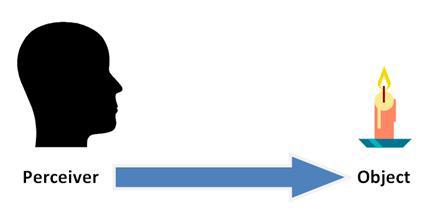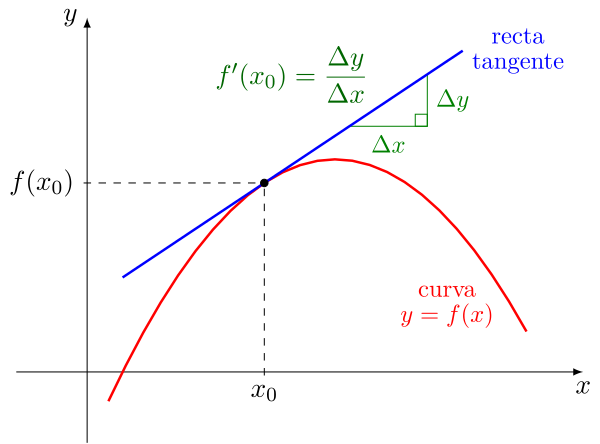# What meanings can we give to philosophical realism, Example of mathematical philosophical realism: The derivativeImage source

There is a reality known to everyone, there is also a reality that cannot be seen and understood by everyone, and there are other humans who are trying to discover other very important realities for humanity, especially the reality that is hidden within science and knowledge. In this entire sense of reality there is a broad philosophical spectrum that allows us to think, investigate and expose our own perspectives about some questions that may arise in a desired interpretation of philosophical realism:

### What is our relationship with reality?

Our relationship with reality may be one that needs to be expanded beyond what we know, the reality we know allows us to stay updated with the knowledge that is already discovered, that is, we will be able to perform based on the basic knowledge that is already known, However, when we manage to get involved with the human needs that drive us to discover new knowledge, it is because we are exposing ourselves to a reality that already exists and that only remains to be discovered. To understand this case, it occurs to me to give an example about the concept of infinitesimal calculus associated with differential calculus:

What is the derivative?

It is the value of the slope of a line that is tangent to the curve f(x) at a given point. We can find this definition in any book on infinitesimal calculus, however it has a background that matches the meaning we may have of the mathematical philosophical reality that existed in ancient times when this concept was discovered, which although it already existed under that format of mathematical philosophical reality, was about to be discovered and applied to an academic need of the time, such as the calculation of speed at an instant of time within an interval of position versus time.Image source

We know that if there is an interval where an object has a change of position in a given time, the speed can be calculated, which is nothing more than a slope. However, to calculate it we need the coordinates of the end point and the coordinates of the initial point. . The surrounding reality of this physical condition made us think about the existing need to want to calculate the speed of a graph in the Cartesian plane knowing a single point, that is, to calculate the speed at an instant of time, the problem that arose at that historical moment. was:

How to calculate the speed of a moving object if only one point on the position versus time graph is known?

It was really a substantial problem because to calculate the slope of a line you need two points, the speed is a slope, therefore to calculate it you need the coordinates of the end point and the initial point. Under this need to want to calculate the speed in an instant of time, that is, knowing a single point, is what gives way to discovering mathematical knowledge that was there in existence but to be discovered, so the separation of the two realities makes that there was a before and after:

Before: calculation of the average speed knowing two points.

After: calculation of instantaneous speed knowing two points

The before and after demarcates a known reality in the face of an academic need that is the spearhead to explore a philosophical reality that allows us to conclude that mathematical concepts are there, only to be discovered and used to give an answer to a reality that needs a solution. .

### References

Philosophical realism: meaning and characteristics

Derivative

0
0
0.000